# quantum computers

28. May 2023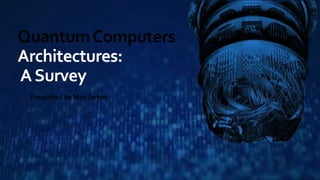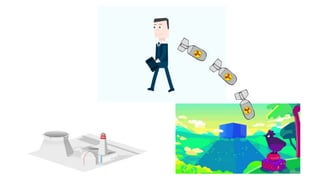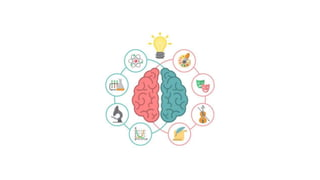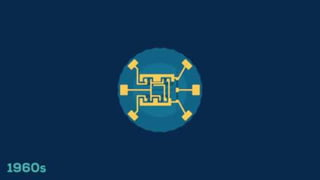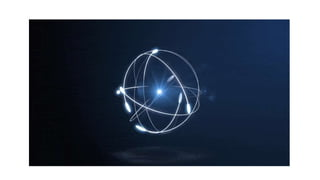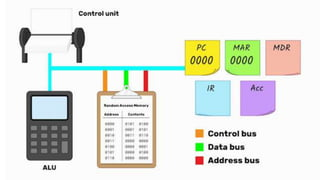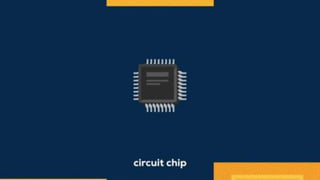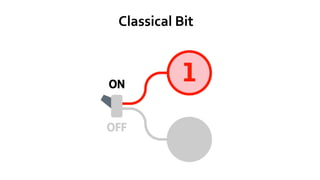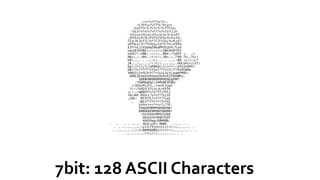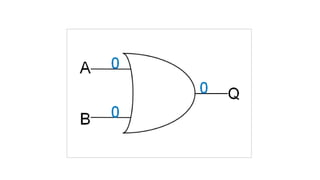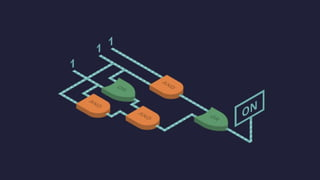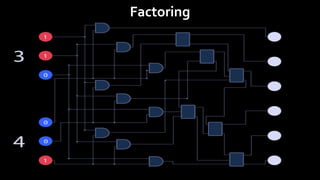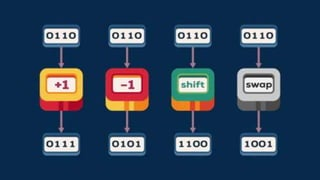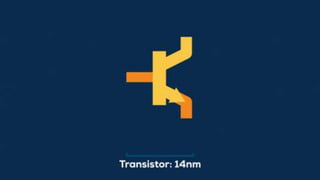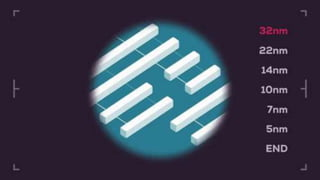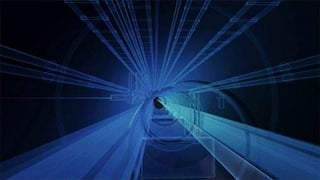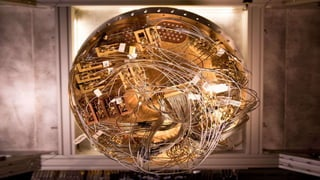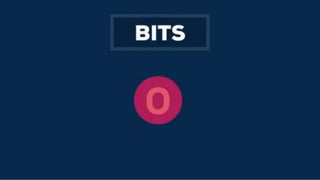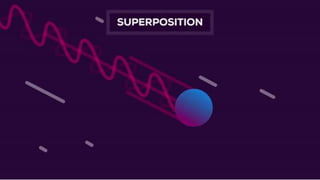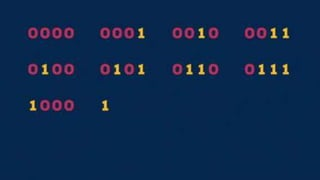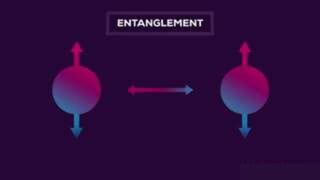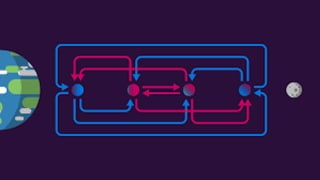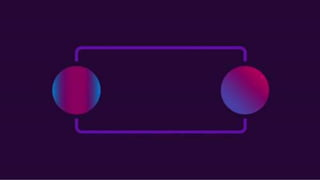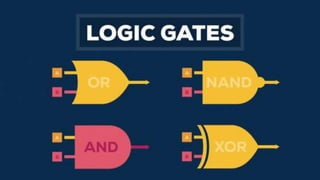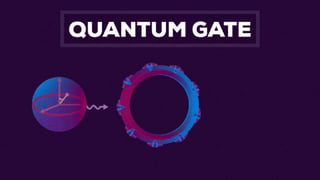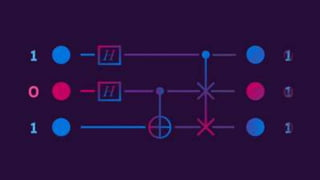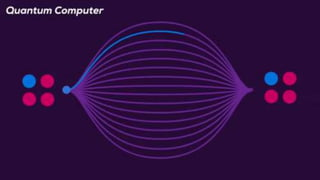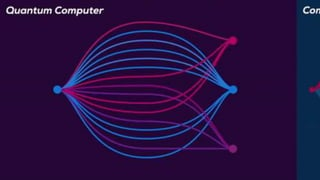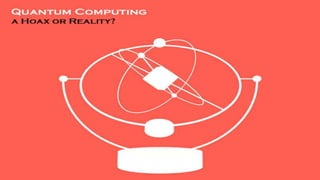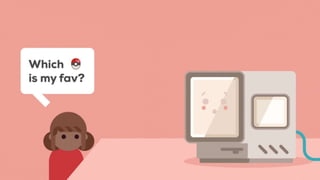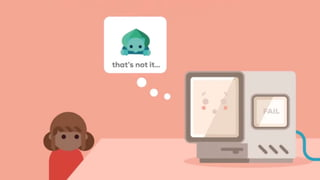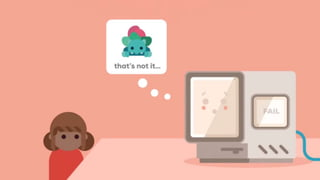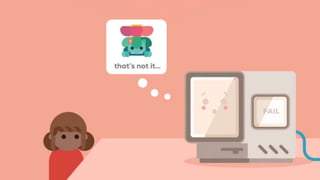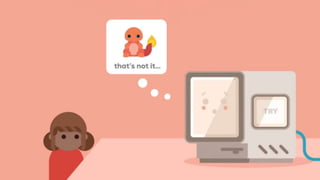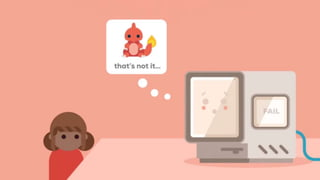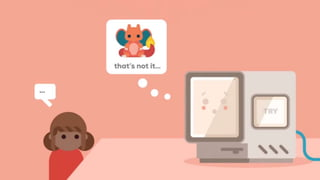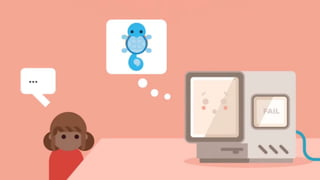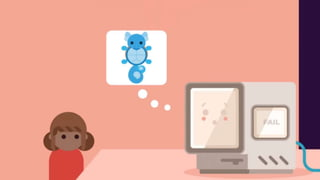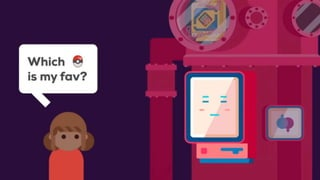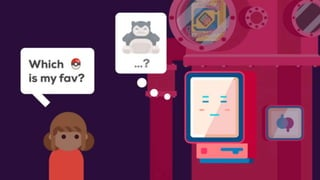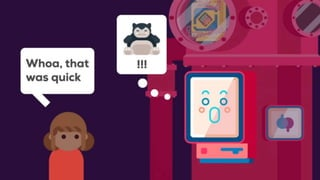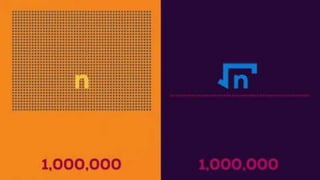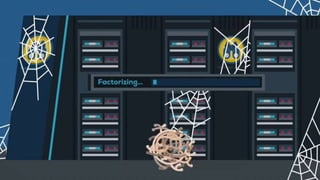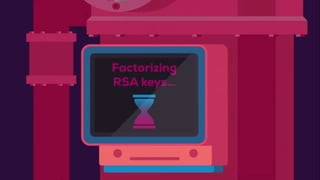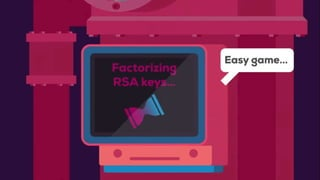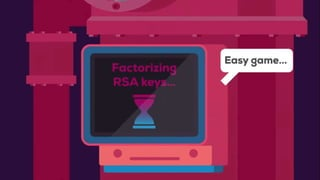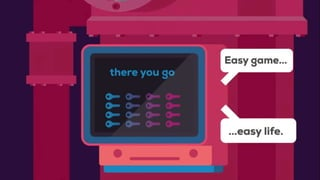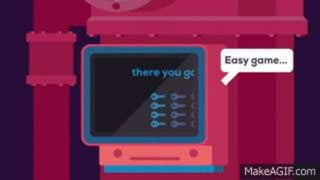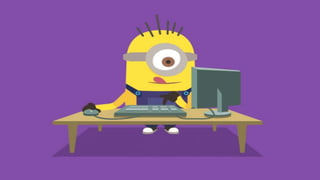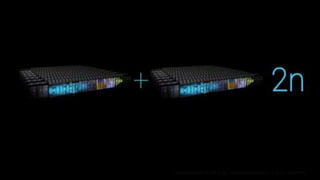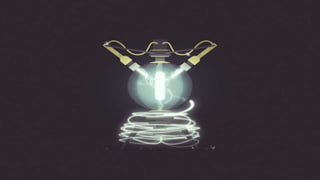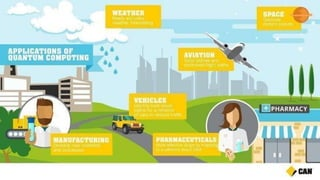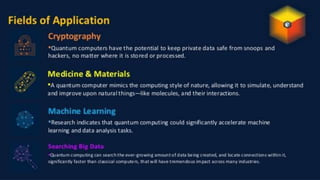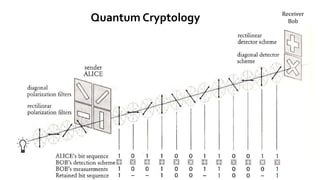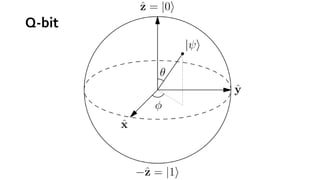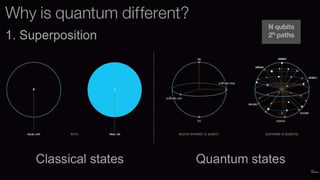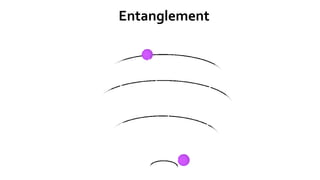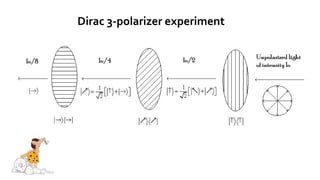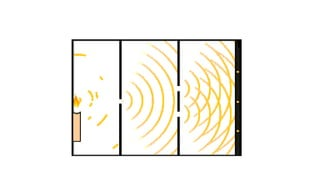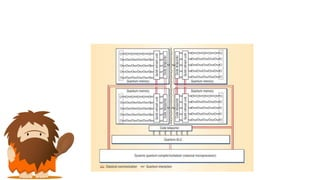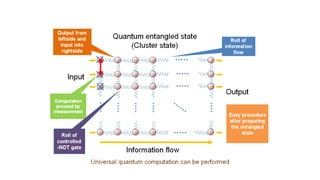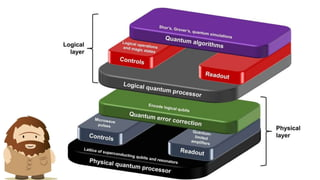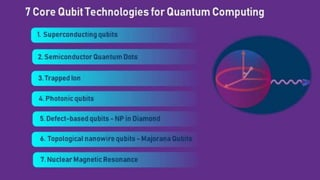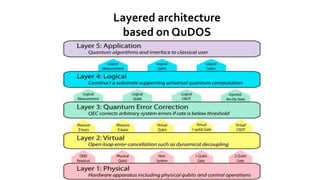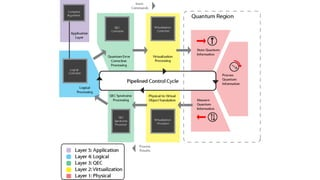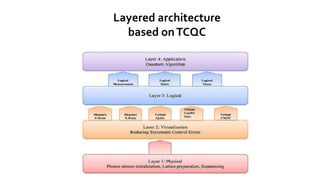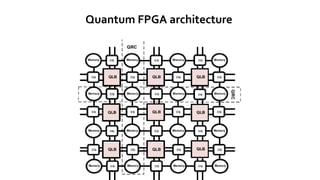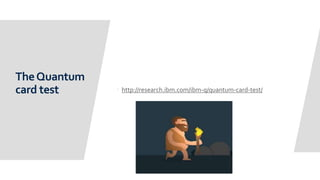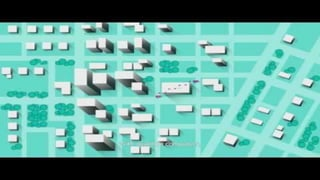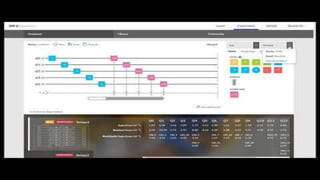1 von 81

### Hinweis der Redaktion

1. For most of our history, human technology consisted of our brains, fire, and sharp sticks.
2. While fire and sharp sticks became power plants and nuclear weapons
3. the biggest upgrade has happened to our brains.
4. Since the 1960's, the power of our brain machines has kept growing exponentially, allowing computers to get smaller and more powerful at the same time. But this process is about to meet its physical limits.
5. Computer parts are approaching the size of an atom.
6. To understand why this is a problem, we have to clear up some basics. A computer is made up of very simple components doing very simple things. Representing data, the means of processing it, and control mechanisms.
7. Computer chips contain modules, which contain logic gates, which contain transistors. A transistor is the simplest form of a data processor in computers, basically a switch that can either block, or open the way for information coming through. This information is made up of bits
8. which can be set to either 0 or 1.
9. Combinations of several bits are used to represent more complex information.
10. Transistors are combined to create logic gates which still do very simple stuff. For example, an AND Gate sends an output of 1 if all of its inputs are 1, and a output of 0 otherwise.
11. Combinations of logic gates finally form meaningful modules, say, for adding two numbers.
12. Once you can add, you can also multiply, and once you can multiply, you can basically do anything.
13. Since all basic operations are literally simpler than first grade math, you can imagine a computer as a group of 7-year-olds answering really basic math questions. A large enough bunch of them could compute anything from astrophysics to Zelda. However, with parts getting tinier and tinier, quantum physics are making things tricky.
14. In a nutshell, a transistor is just an electric switch. Electricity is electrons moving from one place to another. So, a switch is a passage that can block electrons from moving in one direction.
15. Today, a typical scale for transistors is 14 nanometers, which is about 8 times less than the HIV virus' diameter, and 500 times smaller than a red blood cell. As transistors are shrinking to the size of only a few atoms,
16. electrons may just transfer themselves to the other side of a blocked passage via a process called Quantum Tunneling.
17. In the quantum realm, physics works quite differently from the predictable ways we're used to, and traditional computers just stop making sense.
18. We are approaching a real physical barrier for our technological progress.
19. We are approaching a real physical barrier for our technological progress. To solve this problem, scientists are trying to use these unusual quantum properties to their advantage
20. scientists are trying to use these unusual quantum properties to their advantage by building quantum computers.
21. In normal computers, bits are the smallest unit of information. Quantum computers use qubits which can also be set to one of two values.
22. A qubit can be any two level quantum system, such as a spin and a magnetic field, or a single photon.
23. 0 and 1 are this system's possible states, like the photons horizontal or vertical polarization. In the quantum world, the qubit doesn't have to be just one of those, it can be in any proportions of both states at once. This is called superposition. But as soon as you test its value, say, by sending the photon through a filter, it has to decide to be either vertically or horizontally polarized.
24. So as long as it's unobserved, the qubit is in a superposition of probabilities for 0 and 1, and you can't predit which it'll be. But the instant you measure it, it collapses into one of the definite states. Superposition is a game changer.
25. Four classical bits can be in one of two to the power of four different configurations at a time. That's 16 possible combinations, out of which you can use just one.
26. Four qubits in superposition, however, can be in all of those 16 combinations at once. This number grows exponentially with each extra qubit. Twenty of them can already store a million values in parallel.
27. A really weird and unintuitive property qubits can have is Entanglement, a close connection that makes each of the qubits react to a change in the other's state instantaneously,
28. no matter how far they are apart.
29. This means when measuring just one entangled qubit, you can directly deduce properties of it's partners without having to look.
30. Qubit Manipulation is a mind bender as well. A normal logic gate gets a simple set of inputs and produces one definite output.
31. A quantum gate manipulates an input of superpositions, rotates probabilities, and produces another superposition as its output. 4:44
32. So a quantum computer sets up some qubits, applies quantum gates to entangle them and manipulate probabilities, then finally measures the outcome, collapsing superpositions to an actual sequence of 0s and 1s.
33. What this means is that you get the entire lot of calculations that are possible with your setup, all done at the same time.
34. Ultimately, you can only measure one of the results and it'll only probably be the one you want, so you may have to double check and try again.
35. But by cleverly exploiting superposition and entanglement, this can be exponentially more efficient than would ever be possible on a normal computer. So, while quantum computers will not probably not replace our home computers,
36. in some areas, they are vastly superior.
37. One of them is database searching. To find something in a database, a normal computer may have to test every single one of its entries.
38. Quantum computers algorithms need only the square root of that time,
39. which for large databases, is a huge difference The most famous use of quantum computers is ruining IT security. Right now, your browsing, email, and banking data is being kept secure by an encryption system in which you give everyone a public key to encode messages only you can decode. The problem is that this public key can actually be used to calculate your secret private key.
40. Luckily, doing the necessary math on any normal computer would literally take years of trial and error.
41. But a quantum computer with exponential speed-up could do it in a breeze. Another really exciting new use is simulations.
42. Simulations of the quantum world are very intense on resources,
43. and even for bigger structures, such as molecules, they often lack accuracy. So why not simulate quantum physics with actual quantum physics?
44. Quantum simulations could provide new insights on proteins that might revolutionize medicine. Right now, we don't know if quantum computers will be just a specallized tool, or a big revolution for humanity.
45. We have no idea where the limits of technology are,
46. and there's only one way to find out.